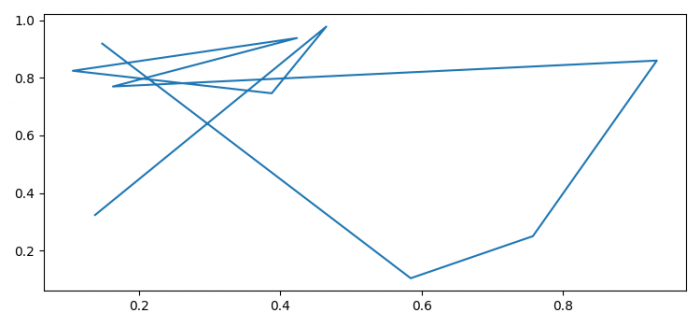# How to disable the keyboard shortcuts in Matplotlib?

To disable the keyboard shortcuts in Matplotlib, we can use remove('s') method.

## Steps

• Set the figure size and adjust the padding between and around the subplots.
• To disable the shortcut "s" to save the figure, use remove("s") method.
• Initialize a variable n for number of data points.
• Create x and y data points using numpy
• Plot x and y data points using plot() method.
• To display the figure, use show() method.

## Example

import numpy as np
from matplotlib import pyplot as plt

plt.rcParams["figure.figsize"] = [7.50, 3.50]
plt.rcParams["figure.autolayout"] = True
plt.rcParams['keymap.save'].remove('s')

n = 10
x = np.random.rand(n)
y = np.random.rand(n)

plt.plot(x, y)

plt.show()

## Output